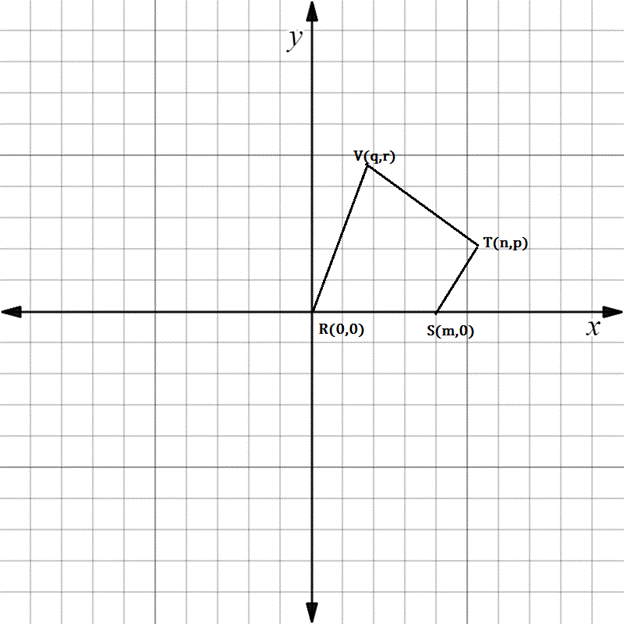Chapter 10.3, Problem 26E### Elementary Geometry for College St...

6th Edition
Daniel C. Alexander + 1 other
ISBN: 9781285195698

#### Solutions

Chapter
Section### Elementary Geometry for College St...

6th Edition
Daniel C. Alexander + 1 other
ISBN: 9781285195698
Textbook Problem
1 views

# In Exercises 23 to 28, find the equation (realtionship) requested. Then eliminate fractions and square root radicals from the equation.For quadrilateral R S T V , suppose that R V - ║ S T - . State an equation that relates m ,   n ,   p ,   q and r .To determine

To state:

An equation that relates m,n, p, q and r for the given figure.

Explanation

The given figure is shown below.

It is given that RVST.

If RV is parallel to ST, then their slopes are equal.

That is mRV=mST

Finding the slope of RV.

x1,y1=R0, 0

x2,y2=Vq, r

Formula for slope of the line containing two points.

Slope is denoted by m.

m=y2-y1x2-x1

Here x1x2

Substituting the x and y co-ordinates.

mRV=r-0q-0

Simplifying,

mRV=rq

Finding the slope of ST

### Still sussing out bartleby?

Check out a sample textbook solution.

See a sample solution

#### The Solution to Your Study Problems

Bartleby provides explanations to thousands of textbook problems written by our experts, many with advanced degrees!

Get Started

#### 63. If

Mathematical Applications for the Management, Life, and Social Sciences

#### The implied domain of is: (1, ∞) (−∞, 1) x ≠ 1 (−1, 1)

Study Guide for Stewart's Single Variable Calculus: Early Transcendentals, 8th

#### Describe how replication protects against fraud being committed in research.

Research Methods for the Behavioral Sciences (MindTap Course List)